# ComplexSISO

Single Input Single Output continuous control block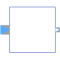# Information

This information is part of the Modelica Standard Library maintained by the Modelica Association.

Block has one continuous Complex input and one continuous Complex output signal.

# Parameters (1)

useConjugateInput Value: false Type: Boolean Description: If true, input is processed conjugate complex

# Connectors (2)

u y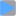Type: ComplexInput Description: Connector of Complex input signal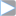Type: ComplexOutput Description: Connector of Complex output signal

# Components (1)

 uInternal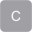Type: Complex Description: Equals either u or conjugate complex input u if useComplexInput = true

# Extended by (15)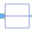ComplexPassThrough Modelica.ComplexBlocks.Routing Pass a Complex signal through without modification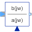TransferFunction Modelica.ComplexBlocks.ComplexMath Complex Transfer Function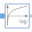Log Modelica.ComplexBlocks.ComplexMath Output the natural (base e) logarithm of the input (input <> '0' required)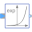Exp Modelica.ComplexBlocks.ComplexMath Output the exponential (base e) of the input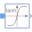Tanh Modelica.ComplexBlocks.ComplexMath Output the hyperbolic tangent of the input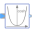Cosh Modelica.ComplexBlocks.ComplexMath Output the hyperbolic cosine of the input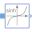Sinh Modelica.ComplexBlocks.ComplexMath Output the hyperbolic sine of the input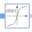Atan Modelica.ComplexBlocks.ComplexMath Output the arc tangent of the input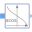Acos Modelica.ComplexBlocks.ComplexMath Output the arc cosine of the input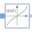Asin Modelica.ComplexBlocks.ComplexMath Output the arc sine of the input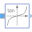Tan Modelica.ComplexBlocks.ComplexMath Output the tangent of the input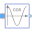Cos Modelica.ComplexBlocks.ComplexMath Output the cosine of the input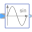Sin Modelica.ComplexBlocks.ComplexMath Output the sine of the input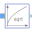Sqrt Modelica.ComplexBlocks.ComplexMath Output the square root of the input (= principal square root of complex number)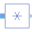Conj Modelica.ComplexBlocks.ComplexMath Output is equal to the conjugate complex input signal